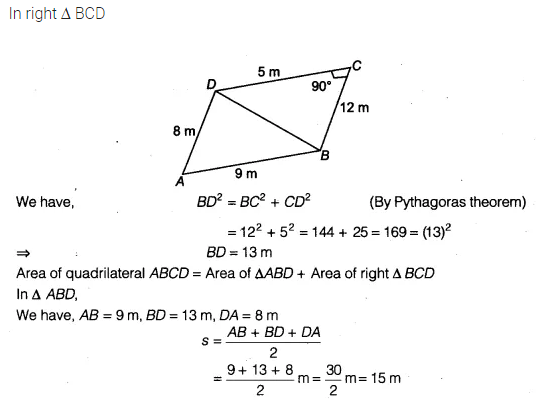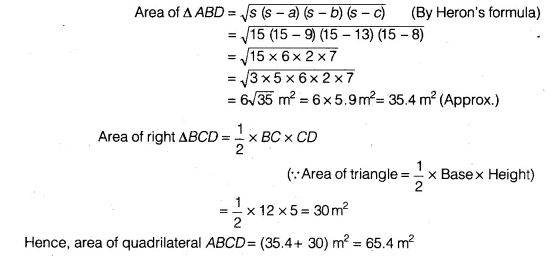# A park, in the shape of a quadrilateral ABCD, has ∠C = 90°, AB = 9m,BC = 12m,CD = 5m and AD = 8 m

A park, in the shape of a quadrilateral ABCD, has ∠C = 90°, AB = 9m,BC = 12m,CD = 5m and AD = 8 m. How much area does it occupy?